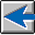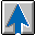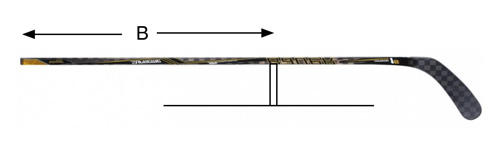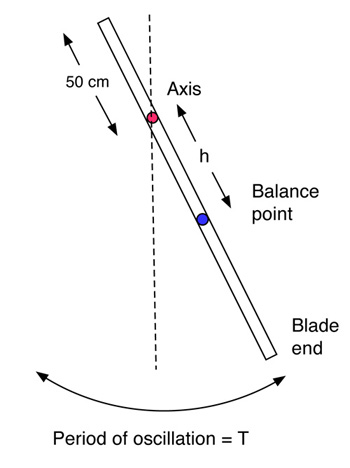Swingweight of Hockey Sticks

Rod Cross, University of Sydney, Sydney, Australia
Crawford Lindsey, Tennis Warehouse, San Luis Obispo, CA
November 15, 2016

#### 1. Swingweight

The weight of a hockey stick is important to a player, and so is its weight distribution. If most of the weight was up at the handle end (as it is in a billiards cue) then the player would have a hard time hitting the puck with any force. If most of the weight was at the blade end (as it is in golf club or a baseball bat), then the blade would be hard to maneuver. In practice, the blade end is heavier than the handle end, the balance point being located about 92 cm from the handle end in most sticks.

The balance point is the point where the stick can be balanced in a horizontal position when the shaft is placed on a thin, vertical edge, as shown in Fig. 1. The balance point means the same thing as the center of mass, but it refers more specifically to the distance from the end of the handle to the center of mass. In theory, there are many different ways that the balance point could be located 92 cm from the handle end. For example, most of the weight could be located at the extreme ends, with very little weight along the shaft itself. Such as a stick would be very fragile, so for practical reasons the weight is distributed more or less evenly along the whole shaft. Nevertheless, not all sticks have the same weight distribution since some are thinner or wider in the tapered section near the blade and since not all blades or handles have the same weight.Figure 1 — The balance point is at a distance B from the handle end, where the stick can be balanced on a thin, vertical edge.

Any two sticks with the same balance point are likely to have a slightly different weight distribution. Not radically different, but enough to have an effect on the swingweight of the stick. The swingweight is not the same thing as the weight of the stick. The weight determines the force needed to lift the stick or to accelerate it in a straight line. The swingweight determines the force needed to swing the stick or to accelerate it in a circular arc. When accelerating a stick or any other object in a straight line, it is often convenient to push or pull it in line with its center of mass. That way, it will move in a straight line without rotating. When swinging a hockey stick, the player pulls the handle with the top hand and pushes the shaft with the bottom hand. As a result, the stick moves forward and it also rotates. The forward speed or acceleration depends on the total force on the stick and its weight. The rotation speed depends on the applied torque and on the swingweight.

The swingweight itself depends on the weight of the stick and its length and it weight distribution. It also depends on which axis it is rotating about, so it is more complicated than ordinary weight. In general, the swingweight increases when the weight increases, it increases when the length increases, it increases when weight is shifted further away from the rotation axis, and it increases when the axis moves away from the middle of the stick towards the handle end of the stick. If you want to swing the stick as fast or as quickly as possible then it helps to use a stick with a low swingweight. For example, a very light or a very short stick can be swung faster than a heavy or a long stick since it will have a lower swingweight. However, sticks with a low swingweight tend to be less powerful than sticks with a large swingweight.

#### 2. Measurement of Swingwieght

It easy to measure the weight and the balance point of a stick. A measurement of swingweight is more complicated, since it is necessary to swing the stick. Swinging a stick is important when hitting a puck, and it is just as important when measuring how the stick performs. A simple arrangement is shown in Fig. 2 where the stick is swung about a fixed axis near the top end of the stick. If pulled aside and released, it will swing back and forth like a pendulum. It is necessary only to measure the period of oscillation, T, and the distance, h, from the axis to the balance point. The period can be measured with a stopwatch or by filming the oscillations with a video camera. Any axis can be chosen, although the measured swingweight depends on the chosen axis. Nevertheless, once the swingweight is measured for that axis, it is easy to calculate the swingweight for any other axis.Figure 2 — The swingweight of a hockey stick can be measured by swinging it as a pendulum.

The formula for the swingweight, IA in kg x m2, about the chosen axis is

(1)   IA = MghT2 / 4π2

where M is the mass of the stick in kg, g = 9.8m/s2 is the acceleration of gravity, h is measured in meters, T is measured in seconds, and π = 3.14.

We measured the swingweight for 13 high performance sticks this way, using an axis located 50 cm from the end of the handle. In every case we found that T was between 2.03 and 2.10 seconds. For a stick with M = 0.42 kg, h = 0.42 m, and T = 2.03 s, the above formula gives IA = 0.180 kg x m2 or 1800 kg x cm2.

When a player swings a stick, the swing axis is not necessarily 50 cm from the handle end. Suppose that the axis is 30 cm from the handle end. Calculations show that the swingweight will then be about 0.280 kg x m2 or 2800 kg x cm2 on average for the sticks in the above table.

If the stick is swung about an axis passing through its balance point, then the swingweight will have a value IB. If the stick is swung about a different axis, located a distance h from the balance point, then the swingweight is given by IA = IB + Mh2. A measurement of IA can therefore be used to calculate IB. Once IB is determined, then the swingweight can be calculated for any other axis located at any other distance h from the balance point.

Stick Brand Model Mass(g) Balance(cm) Swingweight(kg x cm2) Table 1Mass, Balance Point and Swingweight of 13 Sticks 1 Bauer Supreme 18 438 92 1890 2 Bauer Nexus 1N 444.4 91.2 1881 3 Bauer Vapor 1X 422.8 89.2 1703 4 Sher-wood Rekker EK60 400.3 95.7 1879 5 Sher-wood BPM 150 434.8 95.3 2158 6 Sher-wood BPM 90 493.9 93.8 2222 7 Warrier Covert QRL 424.8 92.1 1837 8 True XCore 9 441.6 92.4 1987 9 True A6.0 SBP 411.6 94.6 1948 10 CCM RibCor Trigger 433.1 94 1958 11 CCM Super Tacks 429.6 92.6 1942 12 CCM RBZ Revolution 440.2 94.3 2003 13 STX Surgeon RX2 428.3 93.0 1892

Table 1 — Mass, balance and swingweight of 13 hockey sticks.